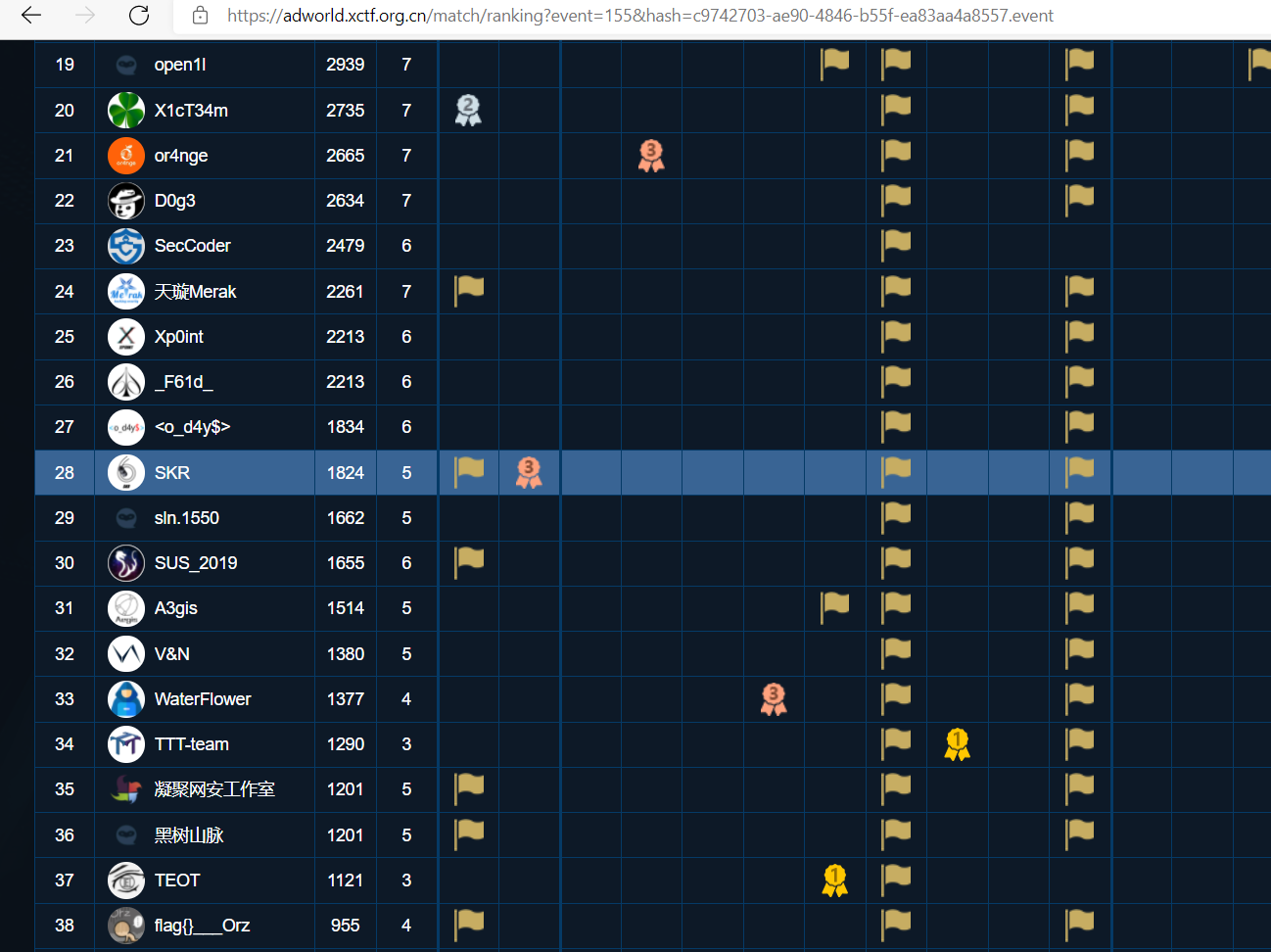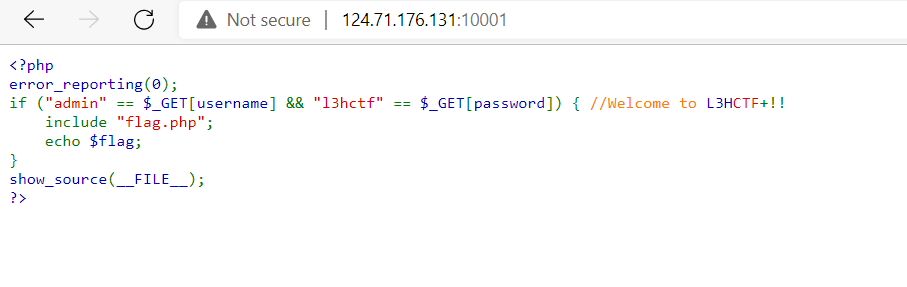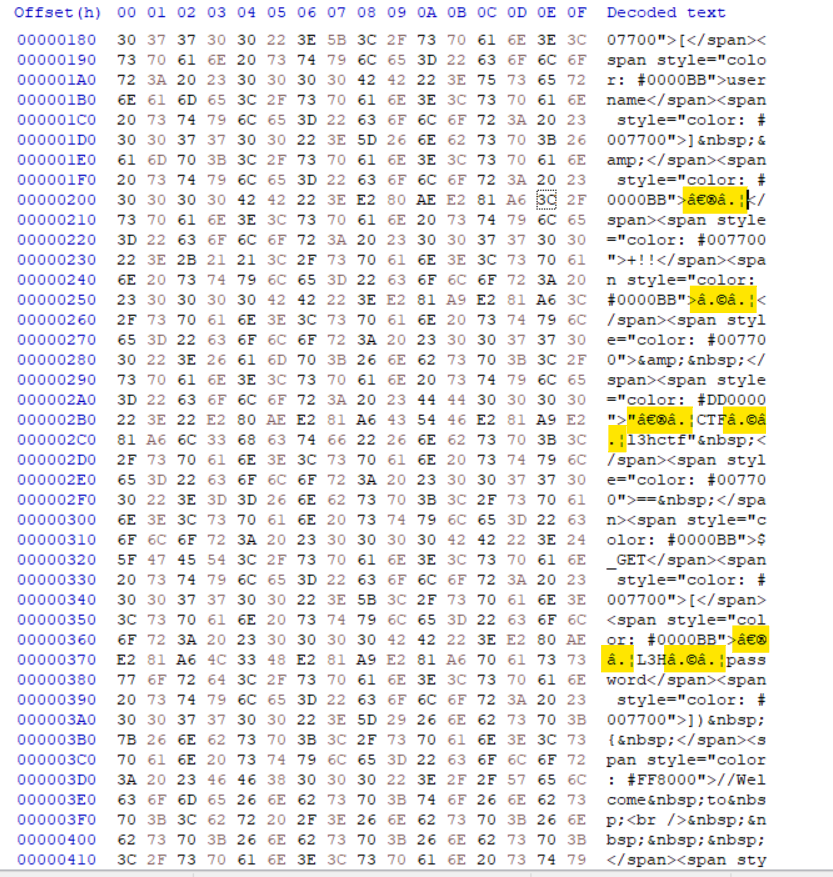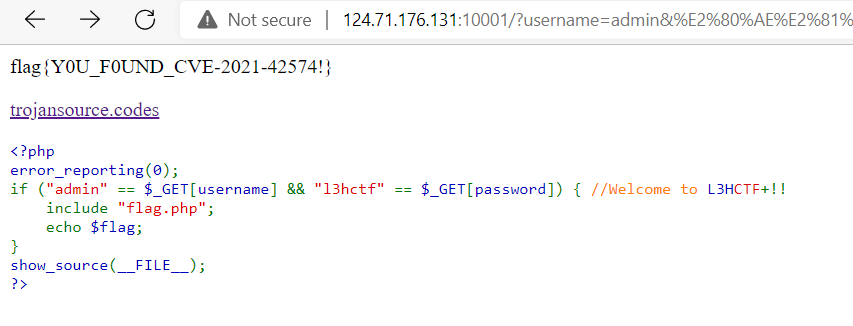We participate L3HCTF last week, it was hosted by L3H_Sec, if interested can try it out at this link: https://l3hctf.xctf.org.cn/

We managed to get 28th place!Here are some challenges I think it is interesting

# Easy PHP

Challenge Description

``````RGB!
http://124.71.176.131:10001/
``````

Goto the link it show us the source:As you can see, obviously we need to pass in `username=admin` and `password=l3ctf` as GET parameter to get the flag!

But I tried `http://124.71.176.131:10001/?username=admin&password=l3hctf` it shows nothing =(

Notice something strange when I copy the source code:

``````<?php
error_reporting(0);
include "flag.php";
echo \$flag;
}
show_source(__FILE__);
?>
``````

See that? Some characters in the comment move in the source code!

Then I download the source and view it in hex editor, saw some weird unicode in the code:Copy the parameter directly from the source code can get the real value

That means the parameter should be:

``````username=admin

``````

Goto `http://124.71.176.131:10001/?username=admin&%E2%80%AE%E2%81%A6L3H%E2%81%A9%E2%81%A6password=%E2%80%AE%E2%81%A6CTF%E2%81%A9%E2%81%A6l3hctf` will get the flag!## Flag

``````flag{Y0U_F0UND_CVE-2021-42574!}
``````

# EzECDSA

## Challenge Description

``````A simple ECDSA.
nc 121.36.197.254 9999
``````

## Challenge files

We are given a netcat service and a python source code

Try netcat to it:

``````nc 121.36.197.254 9999
sha256(XXXX+YC1bIyPhB4EmdIW2) == 730f3c43eb757fb88a3c9b1ee63b987b9b055ac892f3b6412c321a5d83a547ab
Give me XXXX:
``````

Look like we need to brute force some hashes

After looking the source code, that actually just a proof of work, real challenge go after that:

``````def handle(self):
try:
if not self.proof_of_work():
return

dA = random.randrange(n)
Public_key = mul(dA, G)
self.dosend(str(Public_key).encode() + b'\n')

for _ in range(100):
msg = self.recvall(100)
hash = _sha256(msg.encode())
k = random.randrange(n)
kp = k % (2 ** kbits)
P = mul(k, G)
r_sig = P
k_inv = gmpy2.invert(k, n)
s_sig = (k_inv * (hash + r_sig * dA)) % n
self.dosend(b"r = " + str(r_sig).encode() + b'\n')
self.dosend(b"s = " + str(s_sig).encode() + b'\n')
self.dosend(b"kp = " + str(kp).encode() + b'\n')
self.dosend(b"hash = " + str(hash).encode() + b'\n')

self.dosend(b"Give me dA\n")
private_key = self.recvall(300)
if int(private_key) == dA:
self.dosend(FLAG)

except:
self.dosend(b"Something error!\n")
self.request.close()

``````

ECDSA stand for `Elliptic Curve Digital Signature Algorithm`

After look at the Wikipedia, we know that `r` and `s` is signature pair

And `dA` is the private key (randomly selected)

Basically we can generate 100 signatures and we need to give the `dA` (Private Key) to get the flag

How do we know the private key?

## Research

Notice it leaks `k` last byte everytime we sign:

``````kbits = 8
...
kp = k % (2 ** kbits)
...
self.dosend(b"kp = " + str(kp).encode() + b'\n')
``````

According to wikipedia, `k` has to be secret and different in every signature

After some research, I came across this CTF writeup, which quite similar to this challenge

It is called ECDSA leaked nonce

There is many exploit online, I use this nice python code in github to exploit this: https://github.com/bitlogik/lattice-attack

## Exploit

Firstly, I use pwntools to brute force the proof of work:

``````key = pwnlib.util.iters.mbruteforce(lambda x: hashlib.sha256(x.encode()+text).hexdigest() == h.decode(), string.ascii_letters+string.digits, length = 4)
p.sendlineafter("Give me XXXX:",key)
``````

Then get the public key, and generate 100 signatures, then collect all in json format:

``````sigs = []
p.recvuntil('(')
public_key = p.recvuntil(')')[:-1].split(b', ')

# Collect all 100 signatures data
for i in range(100):
result = p.recvuntil("Give me").split(b'\n')
r = int(result.split(b" = "))
s = int(result.split(b" = "))
kp = int(result.split(b" = "))
h = int(result.split(b" = "))
sigs.append({
"r":r,
"s":s,
"kp":kp
})
if i != 99:
p.sendline('a')

data = {
"curve": "SECP256K1",
"public_key": [int(public_key), int(public_key)],
"known_type": "LSB",
"known_bits": 8,
"signatures": sigs,
# All message is 'a'
"message": 
}
# Put the data in data.json
with open("data.json", "w") as fout:
json.dump(data, fout)
p.interactive()
``````

Run it!! Full python script

``````python3 solve.py
[+] Opening connection to 121.36.197.254 on port 9999: Done
solve.py:7: BytesWarning: Text is not bytes; assuming ASCII, no guarantees. See https://docs.pwntools.com/#bytes
p.recvuntil("sha256(XXXX+")
solve.py:8: BytesWarning: Text is not bytes; assuming ASCII, no guarantees. See https://docs.pwntools.com/#bytes
text = p.recvuntil(") == ")[:-5]
solve.py:9: BytesWarning: Text is not bytes; assuming ASCII, no guarantees. See https://docs.pwntools.com/#bytes
h = p.recvuntil("\n")[:-1]
[+] MBruteforcing: Found key: "NbmC"
solve.py:13: BytesWarning: Text is not bytes; assuming ASCII, no guarantees. See https://docs.pwntools.com/#bytes
p.sendlineafter("Give me XXXX:",key)
/home/hong/.local/lib/python3.8/site-packages/pwnlib/tubes/tube.py:822: BytesWarning: Text is not bytes; assuming ASCII, no guarantees. See https://docs.pwntools.com/#bytes
res = self.recvuntil(delim, timeout=timeout)
solve.py:15: BytesWarning: Text is not bytes; assuming ASCII, no guarantees. See https://docs.pwntools.com/#bytes
p.recvuntil('(')
solve.py:16: BytesWarning: Text is not bytes; assuming ASCII, no guarantees. See https://docs.pwntools.com/#bytes
public_key = p.recvuntil(')')[:-1].split(b', ')
solve.py:18: BytesWarning: Text is not bytes; assuming ASCII, no guarantees. See https://docs.pwntools.com/#bytes
solve.py:20: BytesWarning: Text is not bytes; assuming ASCII, no guarantees. See https://docs.pwntools.com/#bytes
result = p.recvuntil("Give me").split(b'\n')
solve.py:32: BytesWarning: Text is not bytes; assuming ASCII, no guarantees. See https://docs.pwntools.com/#bytes
p.sendline('a')
[*] Switching to interactive mode
dA
\$
``````

After it turn into interactive mode, run the github code with the json file to calculate the private key:

``````python3 lattice_attack.py -f ../data.json

----- Lattice ECDSA Attack -----
Running with 8 bits of k (LSB)
Starting recovery attack (curve SECP256K1)
Constructing matrix
Solving matrix ...
LLL reduction
Key found \o/
0xd3064c5df4c50a2c2646d70c98a3a8844b35965ed50be57cc7aa3716e4f3dcb6
``````

Convert the key to decimal, submit it and get the flag!!

`````` 95449139199232530993693727845694023553263076160336750984985659887133098499254
L3HCTF{c7b7e21f60fd1e2deb233fcfd7ebfa12}[*] Got EOF while reading in interactive
``````

## Flag

``````L3HCTF{c7b7e21f60fd1e2deb233fcfd7ebfa12}
``````